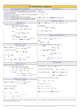How to Learn in 24 Hours?The Rapid Learning Movie

 Need Help? M-F: 9am-5pm(PST): Toll-Free: (877) RAPID-10 US Direct: (714) 692-2900 Int'l: 001-714-692-2900 24/7 Online Technical Support: The Rapid Support Center Secure Online Order:Got Questions? Frequently Asked Questions
 Need Proof? Testimonials by Our Users
 Trustlink is a Better Business Bureau Program. Rapid Learning Center is a fivr-star business. External TrustLink Reviews

 Rapid Learning Courses: MCAT in 24 Hours (2015-16) USMLE in 24 Hours (Boards) Chemistry in 24 Hours Biology in 24 Hours Physics in 24 Hours Mathematics in 24 Hours Psychology in 24 Hours SAT in 24 Hours ACT in 24 Hours AP in 24 Hours CLEP in 24 Hours DAT in 24 Hours (Dental) OAT in 24 Hours (Optometry) PCAT in 24 Hours (Pharmacy) Nursing Entrance Exams Certification in 24 Hours eBook - Survival Kits Audiobooks (MP3)

 Tell-A-Friend: Have friends taking science and math courses too? Tell them about our rapid learning system.

 FAIL (the browser should render some flash content, not this).Home »  Mathematics »  Trigonometry

Solving Trigonometric Equations

 Topic Review on "Title": Trigonometric equation: An equation consisting of trigonometric functions.  Solutions of trigonometric equations: The values of x where the trigonometric equations are defined within the domain of the trigonometric functions.

Rapid Study Kit for "Title":
 Flash Movie Flash Game Flash Card Core Concept Tutorial Problem Solving Drill Review Cheat Sheet"Title" Tutorial Summary : Solving trigonometric equations is discussed in this tutorial with help of examples and identities. Simplification principles are used to make the solution process much easier and manageable. Different types of trigonometric equations are presented with the use of examples and visual aids. Solving trigonometric equations requires simplification techniques and use of algebra because both principles are related.

 Tutorial Features: Specific Tutorial Features: • Step by step solving of trigonometric equations. • Examples of the type of trigonometric equations that are encountered in a typical course. Series Features: • Concept map showing inter-connections of new concepts in this tutorial and those previously introduced. • Definition slides introduce terms as they are needed. • Visual representation of concepts • Animated examples—worked out step by step • A concise summary is given at the conclusion of the tutorial.

 "Title" Topic List: Definition of a trigonometric equation Solving trigonometric equations Type sin x=a Type cos x=a Type tan x= a Type sin x=sin a Type cos x= cos a Type tan x =tan a Type a cosx +b sin x=c

See all 24 lessons in Trigonometry, including concept tutorials, problem drills and cheat sheets:
Teach Yourself Trigonometry Visually in 24 Hours